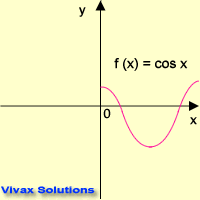# Mathematics

### Odd and Even functions

#### Advanced maths - odd and even functions tutorial

Even Functions

If a function exists such that f(x) = f(-x), it is called an even function.

E.g.f(x) = cos x
This animation shows f(x) = f(-x).
Therefore, f(x) = cos x is an even function.

E.g.

Show that f(x) = 2x4 + x2 is an even function.

f(x) = 2x4 + x2
f(-x) = 2(-x)4 + (-x)2
f(-x) = 2x4 + x2 = f(x)

Therefore, f(x) = 2x4 + x2 is an even function.

Odd Functions

If a function exists such that f(x) = -f(-x), it is called an odd function.

E.g.f(x) =sin x
This animation shows f(x) = -f(-x).
Therefore, f(x) = sin x is an even function.

E.g.

Show that f(x) = x3 + 2x is an odd function.
f(x) = x3 + 2x
f(-x) = (-x)3 + 2(-x) = -x3 -2x
-f(-x) = x3 + 2x = f(x)

Therefore, f(x) = x3 + 2x is an odd function.

Please work out the following questions to complement what you have just learnt.

1. Show by drawing or otherwise that f(x) = 1/x and f(x) = x3 are odd functions.
2. Show that f(x) = (x - 2)(x + 2)/(x2 + 2) is an even function.
3. Check whether f(x) = x3 / (x2 + 7) is odd or even.
4. Show that f(x) = (x3 + x) /(x3 - x) is an even function.Maths is challenging; so is finding the right book. K A Stroud, in this book, cleverly managed to make all the major topics crystal clear with plenty of examples; popularity of the book speak for itself - 7th edition in print.

### Recommended - GCSE & iGCSEThis is the best book available for the new GCSE(9-1) specification and iGCSE: there are plenty of worked examples; a really good collection of problems for practising; every single topic is adequately covered; the topics are organized in a logical order.

### Recommended for A LevelThis is the best book that can be recommended for the new A Level - Edexcel board: it covers every single topic in detail;lots of worked examples; ample problems for practising; beautifully and clearly presented.